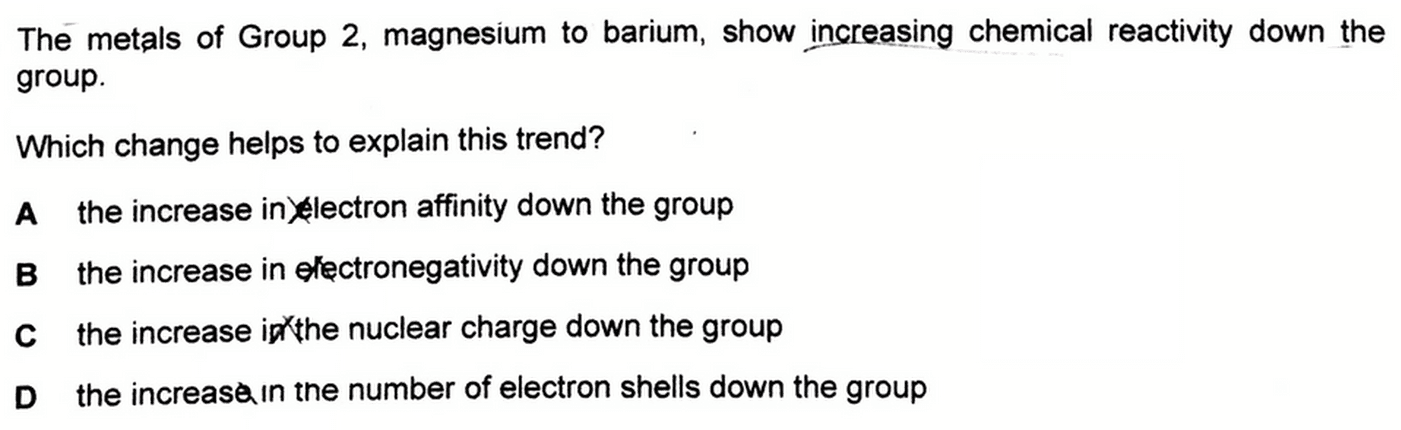# 2019 A Level H2 Chemistry Paper 1 Question 10 - Explain Increasing Chemical Reactivity Down Group 2

Let's take a look at 2019 A Level H2 Chemistry Paper 1 Question 10.Metals only get oxidised by losing their valence electrons.

Therefore the chemical reactivity of metals is equivalent to their ease of oxidation or ease of loss of electrons.

This means that down group 2, it is easier for metals to lose their electrons and get oxidised, hence increasing chemical reactivity.

Let's go through each statement in detail and reason out if it can explain this reactivity trend.

## A. the increase in electron affinity down the groupB. the increase in electronegativity down the group

We can discuss options A and B together, since electron affinity and electronegativity are related.

Electron affinity is how strongly an element can attract valence electrons, while electronegativity is how strongly the element can attract the bonding pair of electrons in a covalent bond.

Down group 2, there is an increase in number of principal quantum shells, hence valence electrons are further away from the nucleus.

This results in a weaker attraction between nucleus and valence electrons, which means electron affinity and electronegativity will decrease down the group.

In fact electron affinity and electronegativity will decrease down any group in the Periodic Table.

Hence A and B are false and not the answer.

## C. the increase in the nuclear charge down the group

Down the group there will be more protons in the nucleus hence greater nuclear charge.

However if nuclear charge were the dominant factor in determining reactivity, a greater nuclear charge would mean a stronger attraction between nucleus and valence electrons.

This means it'll be harder to lose electrons, get oxidised, and reactivity should decrease down the group instead.Hence C is true but not the dominant factor to answer this question.

## D. the increase in the number of electron shells down the group

Down the group the number of principal quantum shells increase hence there are more inner shells to block the attraction between nucleus and valence electrons.

Usually down any group, the increase in shielding effect outweighs the increase in nuclear charge effect.

Effective nuclear charge decreases, there is an overall weaker attraction on the valence electrons and less energy is required to remove the electrons.

Therefore ease of oxidation and chemical reactivity increases down group 2.From the above discussion we can conclude the answer to this question is option D.

Topic: Group 2 and 17 Chemistry, Inorganic Chemistry, A Level Chemistry, Singapore

Found this A Level Chemistry video useful?